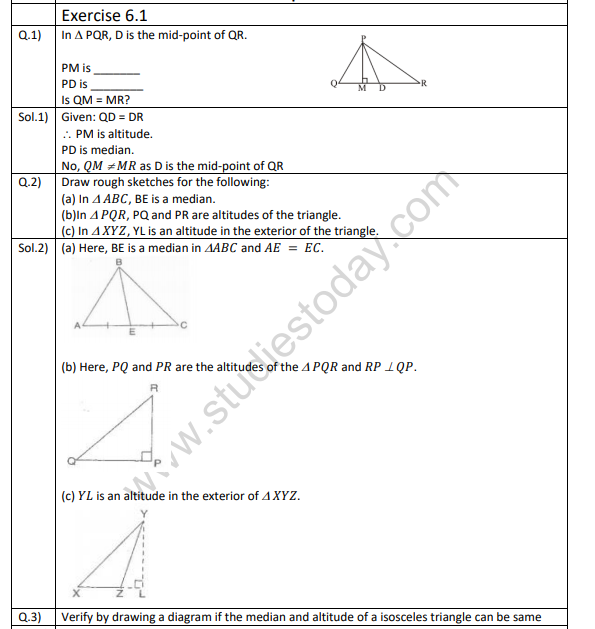# NCERT Solutions Class 7 Mathematics Triangle and its properties

## Triangle and its properties Class 7 NCERT Solutions

Class 7 Mathematics students should refer to the following NCERT questions with answers for Triangle and its properties in standard 7. These NCERT Solutions with answers for Grade 7 Mathematics will come in exams and help you to score good marks

### Triangle and its properties NCERT Solutions Class 7

NCERT Solutions Class 7 Mathematics Triangle and its properties. The NCERT solutions have been made by teacher of one of the best CBSE school in India. These solutions have been made to give detailed answers and expanations of the concepts which can be easily understood by the students. Refer to other links also to download mathematics NCERT solutions, worksheets and test papers.## Books recommended by teachers

 NCERT Solutions Class 7 Mathematics Integers
 NCERT Solutions Class 7 Mathematics Fractions and Decimals
 NCERT Solutions Class 7 Mathematics Data Handling
 NCERT Solutions Class 7 Mathematics Simple Equations
 NCERT Solutions Class 7 Mathematics Lines and angles
 NCERT Solutions Class 7 Mathematics Triangle and its properties
 NCERT Solutions Class 7 Mathematics Congruence of Triangle
 NCERT Solutions Class 7 Mathematics Comparing Quantities
 NCERT Solutions Class 7 Mathematics Rational Numbers
 NCERT Solutions Class 7 Mathematics Practical Geometry
 NCERT Solutions Class 7 Mathematics Perimeter and Area
 NCERT Solutions Class 7 Mathematics Algebraic Expressions
 NCERT Solutions Class 7 Mathematics Exponents and Power
 NCERT Solutions Class 7 Mathematics Symmetry
 NCERT Solutions Class 7 Mathematics Visualizing Solid Shapes